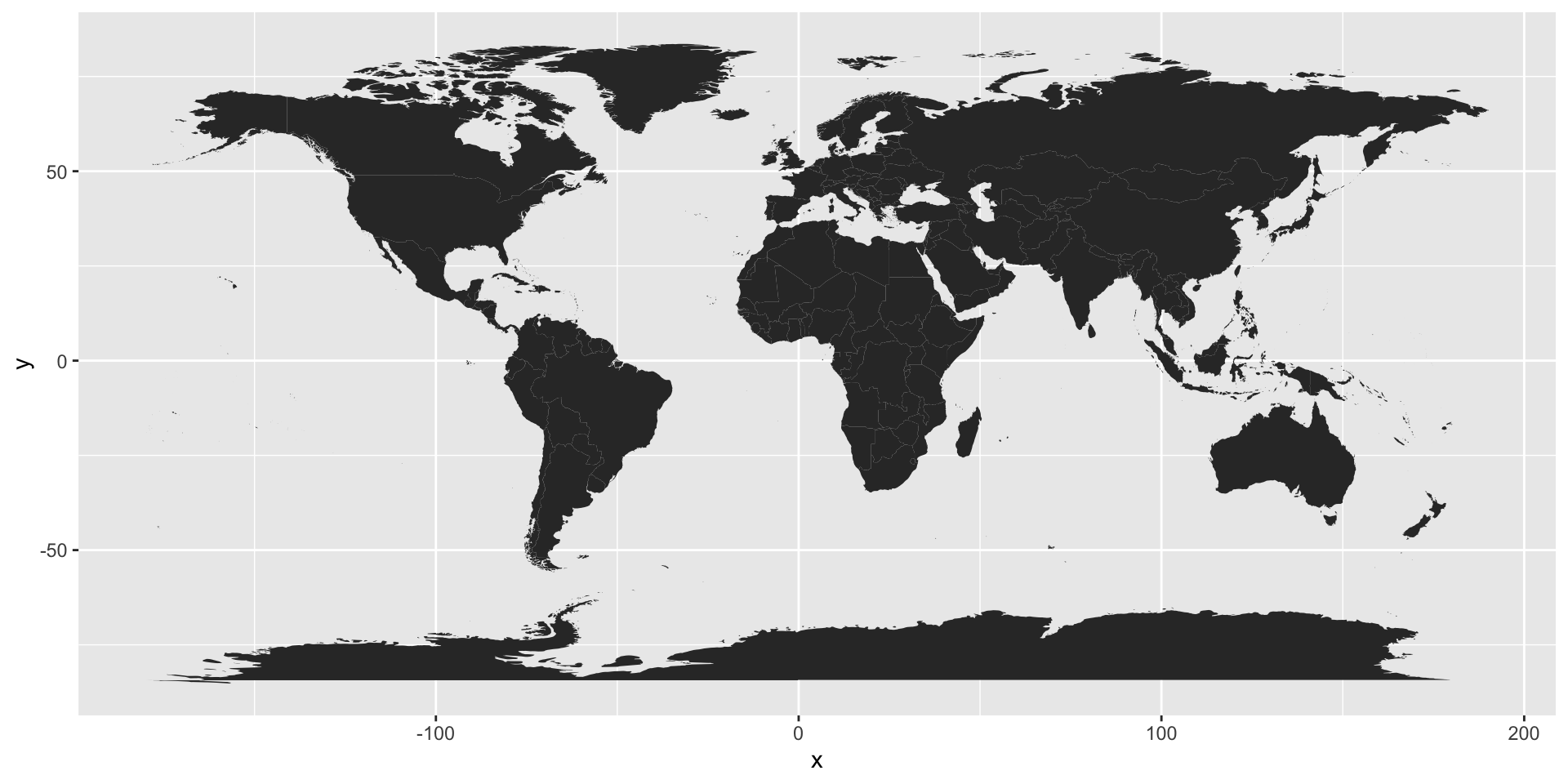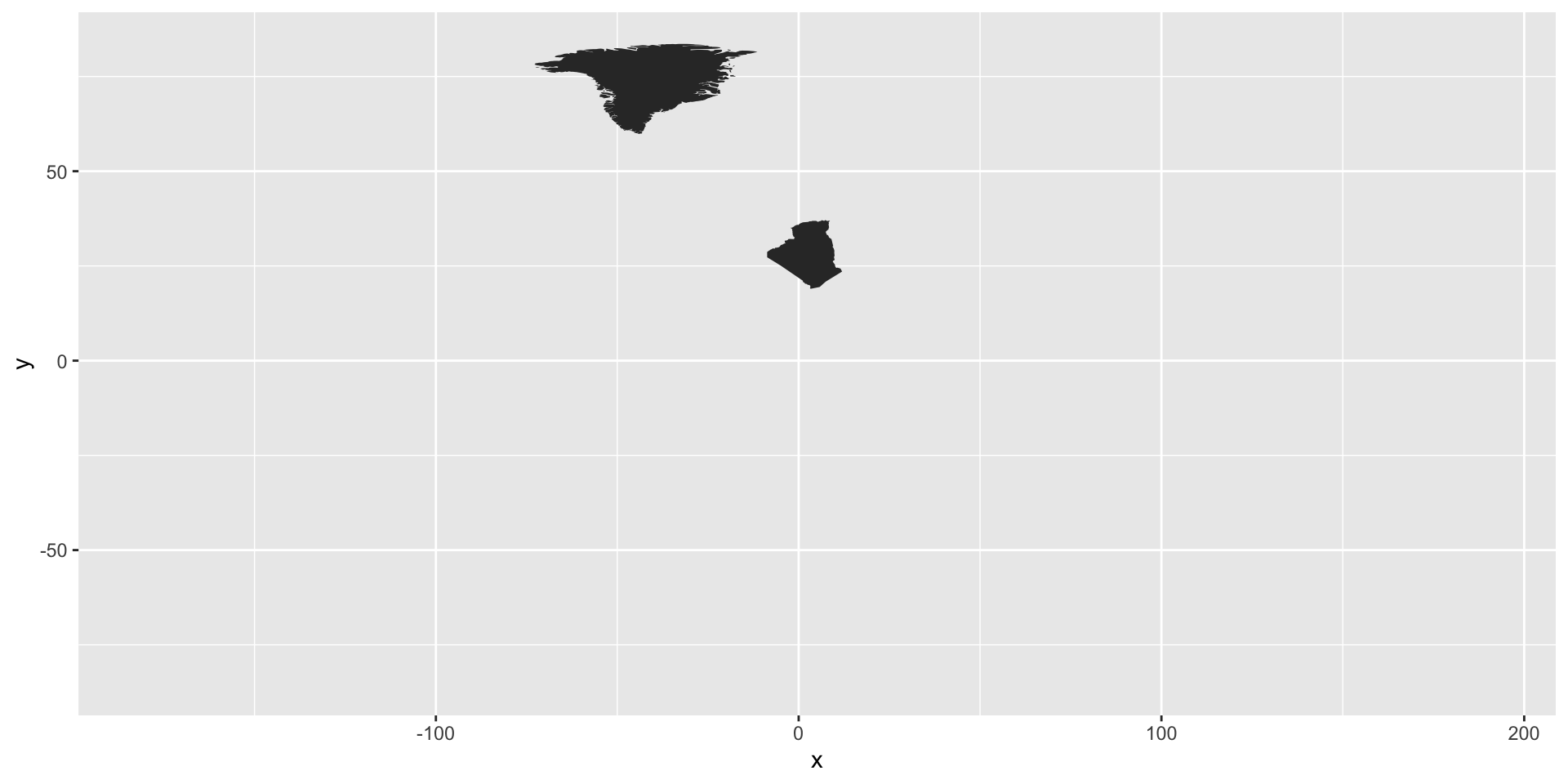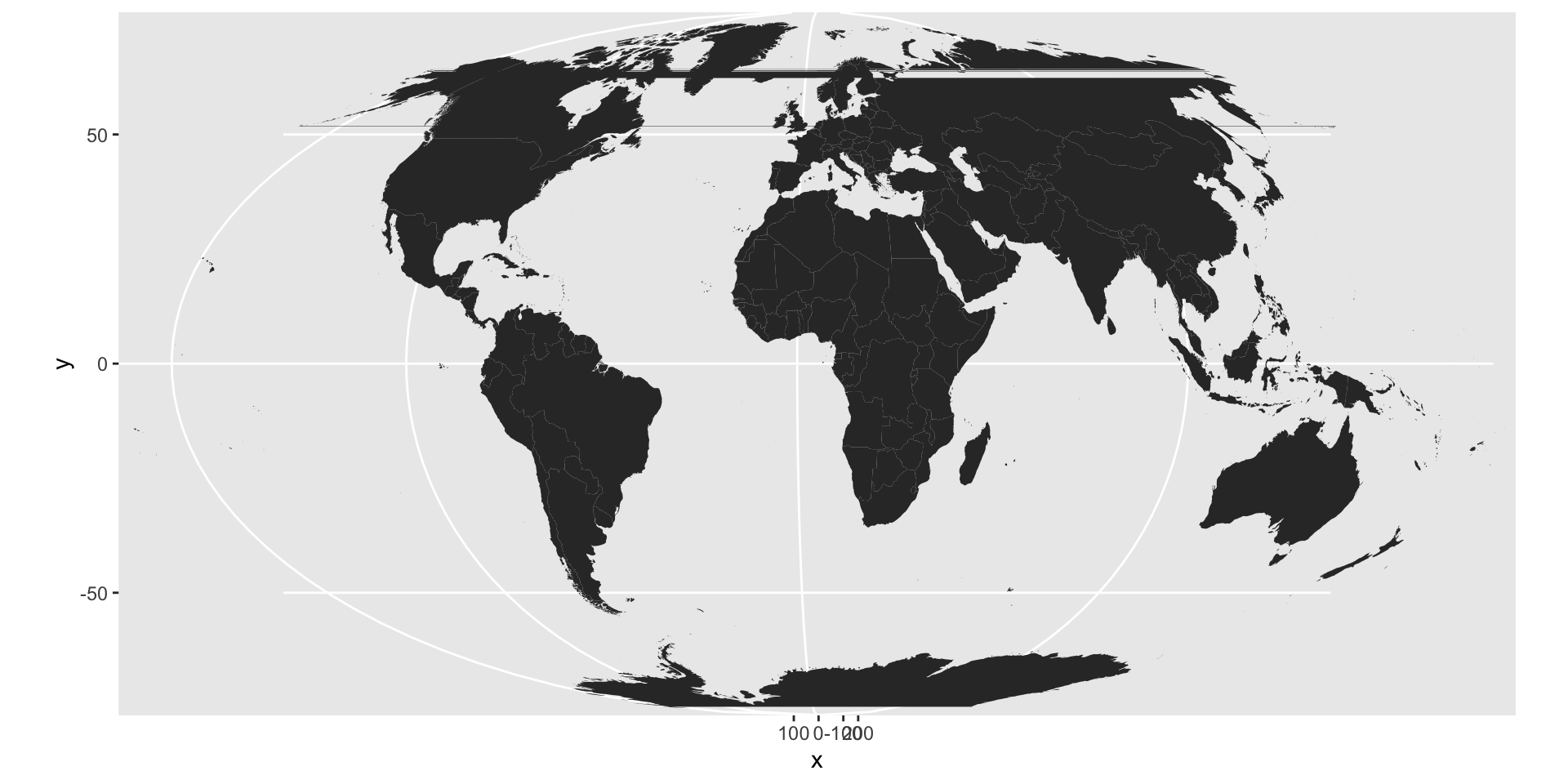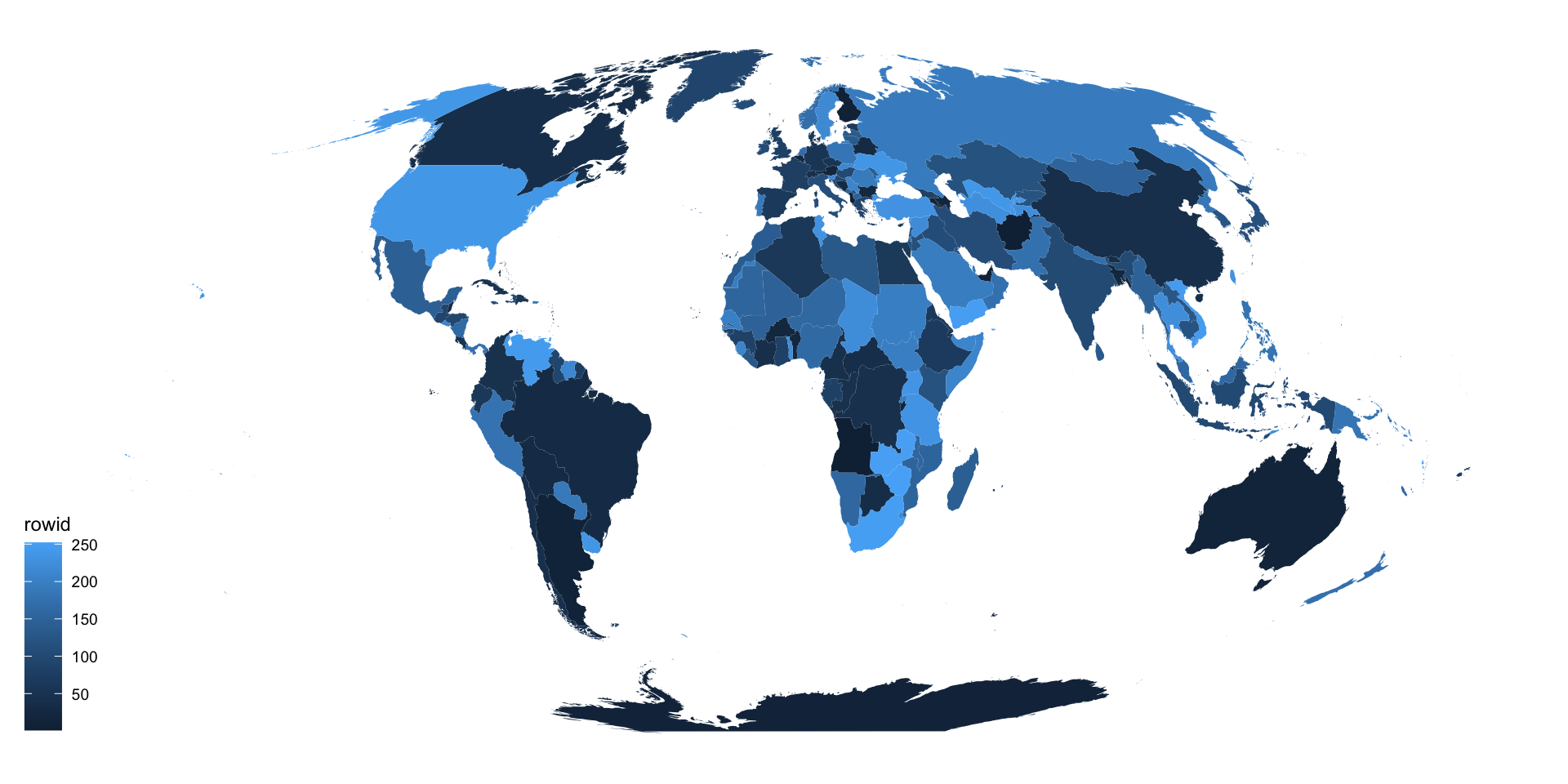There are several different ways to make maps in R, and I always have to look it up and figure this out again from previous examples that I’ve used. Today I had another look at what’s currently possible and what’s an easy way of making a world map in ggplot2 that doesn’t require fetching data from various places.

TLDR: Copy this code to plot a world map using the tidyverse:

``````library(tidyverse)
library(ggthemes)

world_map = map_data("world") %>%
filter(! long > 180)

countries = world_map %>%
distinct(region) %>%
rowid_to_column()

countries %>%
ggplot(aes(fill = rowid, map_id = region)) +
geom_map(map = world_map) +
expand_limits(x = world_map\$long, y = world_map\$lat) +
coord_map("moll") +
theme_map()``````# Explanation

To make a map using `geom_map()` you’ll need two datasets: one that includes the map data (country borders), and another one on how to colour in each area. I tried doing `data = NULL` or `fill = "grey"` to make all countries the same colour, but couldn’t get that to work. So I’m creating the necessary second dataset with `distinct(world_map, region)` which is a list of every country from the map data. This list of countries (=regions) is the first argument to `ggplot()`, the `world_map` data goes inside `geom_map()`. `expand_limits()` are essential as otherwise you will not see anything.

``````library(tidyverse)

# Load map data
world_map = map_data("world")

distinct(world_map, region) %>%
ggplot(aes(map_id = region)) +
geom_map(map = world_map) +
expand_limits(x = world_map\$long, y = world_map\$lat)``````# Equal area projection

Global maps that do not use an equal area projection are a huge pet peeve of mine. The above map is not ‘equal-area’ and the closer to the equator an area is the more diminished it is.

Let’s just plot Greenland and Algeria for example:

``````tibble(region = c("Greenland", "Algeria")) %>%
ggplot(aes(map_id = region)) +
geom_map(map = world_map) +
expand_limits(x = world_map\$long, y = world_map\$lat)``````In reality, Algeria is bigger than Greenland: 2.4 million km2 vs 2.2 million km2!

Therefore, I always use an equal-area projection such as Mollweide:

``````library(tidyverse)

# Load map data
world_map = map_data("world")

distinct(world_map, region) %>%
ggplot(aes(map_id = region)) +
geom_map(map = world_map) +
expand_limits(x = world_map\$long, y = world_map\$lat) +
coord_map("moll")``````But then we get those weird lines in the Northern Hemisphere. That’s because parts of the US and Russia extend across the 180th meridian. And since `geom_map()` is connecting each area with itself it then draws these lines across the globe.

A simple fix I’ve found is to remove map data that has a longitude greater than 180 degrees:

``````library(tidyverse)
library(ggthemes)

world_map = map_data("world") %>%
filter(! long > 180)

countries = world_map %>%
distinct(region) %>%
rowid_to_column()

countries %>%
ggplot(aes(fill = rowid, map_id = region)) +
geom_map(map = world_map) +
expand_limits(x = world_map\$long, y = world_map\$lat) +
coord_map("moll") +
theme_map()``````I’ve also thrown in `theme_map()` from `library(ggthemes)`, put the coutry list which I made with `distinct()` into a separate object, and added in `fill = rowid` for some colour. This is useful as I’m generally plotting chloropleths so I want a tibble with values for each country that I can join with whatever data I have for each country (e.g., student numbers, population, etc.).